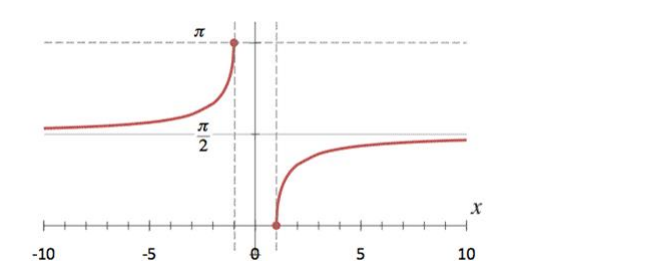# Mark the tick against the correct answer in the following:

Question:

Mark the tick against the correct answer in the following:

Range of $\sec ^{-1} \times$ is

A. $\left[0, \frac{\pi}{2}\right]$

B. $[0, \pi]$

C. $[0, \pi]-\left\{\frac{\pi}{2}\right\}$

D. None of these

Solution:

To Find:The range of $\sec ^{-1}(x)$

Here,the inverse function is given by $\mathrm{y}=\mathrm{f}^{-1}(x)$

The graph of the function $y=\sec ^{-1}(x)$ can be obtained from the graph of

$Y=\sec x$ by interchanging $x$ and $y$ axes.i.e, if $(a, b)$ is a point on $Y=\sec x$ then $(b, a)$ is the point on the function $y=\sec ^{-1}(x)$

Below is the Graph of the range of $\sec ^{-1}(x)$From the graph, it is clear that the range of $\sec ^{-1}(x)$ is restricted to interval

$[0, \pi]-\left\{\frac{\pi}{2}\right\}$